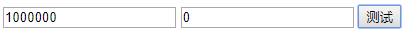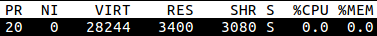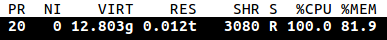版权申明：本文为博主窗户(Colin Cai)原创，欢迎转帖。如要转贴，必须注明原文网址

http://www.cnblogs.com/Colin-Cai/p/11774213.html

作者：窗户

QQ/微信：6679072

E-mail：6679072@qq.com

尾递归

这篇文章，我们讲尾递归。在递归中，如果该函数的递归形式表现在函数返回的时候，则称之为尾递归。

举个简单的例子，用伪码如下：

if a = 0

return b

end

上述代码很容易用循环表示：

while True

if a = 0

return b

end

a <= a-1

b <= b+1

end

end

所有的尾递归都可以用循环表示，只需要把传入的参数当成是状态，运算的过程当成是状态的转换。

3,0

2,1

1,2

0,3

这样的状态转换。

函数的计算会维护一个栈，每当遇到函数调用会记录当前运行的状态，如此在函数返回的时候可以恢复上下文。

比如，对于Fibonacci数列，伪码如下：

function fib(n)

if n < 3

return 1

end

return fib(n-1)+fib(n-2)

end

我们计算fib(4)，栈大致如下：

fib(4)

=>

fib(4)

fib(3)

=>

fib(4)

fib(3)

fib(2)

=>

fib(4)

fib(3)

fib(2) 1

=>

f(4)

f(3) 1+

=>

f(4)

f(3) 1+

f(1)

=>

f(4)

f(3) 1+

f(1) 1

=>

f(4)

f(3) 2

=>

f(4) 2+

=>

f(4) 2+

f(2)

=>

f(4) 2+

f(2) 1

=>

f(4) 3

=>

3

=>

=>

=>

=>

=>

=>

=>

=>

3

这里，可以采用一个编译技术，就是尾递归优化，其一般情况是，如果一个函数的计算中遇到了完全转化成另一个函数调用的情况，那么栈的当前函数部分的信息可以完全抹去，而替换为新的函数。如此处理下，此情况栈不会增长。

=>

=>

=>

=>

3

尾递归优化给了我们一种迭代的方式，之所以研究它，在于函数式编程会用到它。

注：递归论区分递归和迭代(迭置)，和计算机上定义有一点区别，在此不深入。

C/C++

我们从底层的语言开始，首先还是上面的加法实现。为了让范围更大一点，便于观察，我们使用unsigned long long类型。

/*add.c*/
unsigned long long add(unsigned long long a, unsigned long long b)
{
if(a==0ULL)
return b;
}

#include <stdio.h>
unsigned long long add(unsigned long long a, unsigned long long b);
int main(int argc, char **argv)
{
unsigned long long a, b;
sscanf(argv, "%llu", &a);
sscanf(argv, "%llu", &b);
return 0;
}

用gcc编译，

运行一下，

./a.out 10000000 100000000

马上发生短错误，直接崩溃。看来C语言作为底层语言没必要支持这个啊？

于是我们开启优化，

gcc -O2 add.c main.c -o a.out

然后运行一下

./a.out 10000000000000000 10000000000000000

立即得到我们想要的值而没有发生崩栈

20000000000000000

看来……不对，1亿亿次迭代瞬间完成？

objdump反汇编一把，

00000000004006b0 <add>:
4006b0:       48 8d 04 37             lea    (%rdi,%rsi,1),%rax
4006b4:       c3                      retq   

……原来全被优化了，gcc现在还真强大，直接猜出语义，clang测一把也是如此。

这个并非我们想要的，我们得用其他手段去验证(其实我们可以抽出部分优化选项来，但此处讲的是验证思路)。

此处借助我在《相互递归》中讲的奇偶判断，分三个函数，实现如下，

/*sub1.c*/
unsigned long long sub1(unsigned long long x)
{
return x - 1ULL;
}
/*is_odd.c*/
unsigned long long sub1(unsigned long long x);
int is_even(unsigned long long x);
int is_odd(unsigned long long x)
{
if(x == 0ULL)
return 0;
return is_even(sub1(x));
}
/*is_even.c*/
unsigned long long sub1(unsigned long long x);
int is_odd(unsigned long long x);
int is_even(unsigned long long x)
{
if(x == 0ULL)
return 1;
return is_odd(sub1(x));
}

上述函数是单独编写，甚至，减1的操作也单独用一个文件来实现。如此测试的原因，就在于，我们要排除掉整体优化的可能。

还需要写一个main函数来验证，

/*main.c*/
#include <stdio.h>
int is_odd(unsigned long long x);
int main(int argc, char **argv)
{
unsigned long long x;
sscanf(argv, "%llu", &x);
printf("%llu is %s\n", x, is_odd(x)?"odd":"even");
return 0;
}

以上四个文件单独编译，开启-O2优化选项(当然，其实main无所谓)

for i in sub1.c is_odd.c is_even.c main.c; do gcc -O2 -c $i; done 然后链接， gcc sub1.o is_odd.o is_even.o main.o -o a.out 然后我们对一个很大的数来进行测试， ./a.out 10000000000 一会儿之后，程序打印出 10000000000 is even 以上可以证明，gcc/clang对于尾递归优化支持的挺好。实际上，很早之前大部分C语言编译器就支持了这点，因为从技术上来看，并不是很复杂的事情。而C++也同理。 Python Python实现add如下 def add(a, b): if a==0: return b return add(a-1, b+1) 计算add(1000,0)就崩栈了，显然Python的发行是不支持尾递归优化的。 不过这里栈似乎小了点，可以用sys.setrlimit来修改栈的大小，这实际上是UNIX-like的系统调用。 有人用捕捉异常的方式让其强行支持尾递归，效率当然是损失很多的，不过这个想法倒是很好。想起以前RISC大多不支持奇边界存取值，比如ARM，于是在内核中用中断处理强行支持奇边界错误，虽然效率低了很多，但逻辑上是通过的。异曲同工，的确也是一条路，不过我还是更加期望Python在未来支持尾递归优化吧。 JavaScript 依然是用add测试，编写以下网页 <input type="text" id="in1" /> <input type="text" id="in2" /> <input type="button" id="bt1" onclick="test()" value="测试"/> <script type="text/javascript"> function add(a, b) { if (a==0) { return b; } return add(a-1, b+1); } function test() { a = parseInt(document.getElementById("in1").value); b = parseInt(document.getElementById("in2").value); try { alert(add(a,b)); } catch(err) { alert('Error'); } } </script>就用1000000和0来测试，没看到哪个浏览器不跳出Error的……据说v8引擎做好了，可是人家就不给你用…… Scheme 然后我们来看Scheme，按照Scheme的标准一向强行规定Scheme支持尾递归优化。 我们实现add函数如下 (define (add a b) (if (zero? a) b (add (- a 1) (+ b 1)))) 实现更为复杂的奇偶判断 (define (is-odd x) (if (zero? x) #f (is_even (- x 1)))) (define (is-even x) (if (zero? x) #t (is_odd (- x 1)))) 使用Chez Scheme、Racket、guile测试，使用很大的数来运算， 然后使用top来观测程序的内存使用情况，我们发现，虽然CPU占用率可能是100%，但内存的使用并不增加。就连guile这样的一个小的实现都是如此，从而它们都是符合标准而对尾递归进行优化的。 Common Lisp 测完Scheme，再来测Scheme的本家兄弟，另外一种Lisp——Common Lisp 先用Common Lisp实现add，因为Common Lisp将数据和过程用不同的命名空间，导致代码有点奇怪(似乎很不数学) (defun add(a b) (if (zerop a) b (funcall #'add (- a 1) (+ b 1)))) 使用clisp来运行 (add 10000 10000) 结果就 *** - Program stack overflow. RESET 因为没有尾递归优化的规定，所以对于那种无限循环，Common Lisp只能选择迭代才能保证不崩栈，比如使用do。使用do重新实现add如下 (defun add(a b) (do ((x a (- x 1)) (y b (+ y 1))) ((zerop x) y))) 如此，终于不崩栈了。但是似乎也改变了Lisp的味道，do显然此处只能在设计编译器、解释器的时候就得单独实现，虽然按理Lisp下这些都应该是宏，但是无论用宏如何将函数式编程映射为显示的迭代，因为尾clisp递归优化不支持，则无法和系统提供的do一样。 sbcl是Common Lisp的另外一个实现，在这个实现中，我们使用第一个add函数的版本，没有发生崩栈。我们再来实现一下奇偶判断 (defun is-odd(x) (if (zerop x) '() (funcall #'is-even (- x 1)))) (defun is-even(x) (if (zerop x) t (funcall #'is-odd (- x 1)))) 计算 （is-even 1000000000) 过了几秒，返回了结果t，证明了sbcl对尾递归做了优化。也终于给了我们一个更为靠谱的Common Lisp的实现。 AWK 选择一种脚本语言来测试这个问题，使用GNU awk来实现add awk ' function add(a,b) { if(a==0) return b return add(a-1, b+1) } {print add($1, \$2)}'

运行后，用top来观测内存占用输入

100000000 1

让其做加法内存使用瞬间爆发，直到进程被系统KO。

话说，awk没有对尾递归优化也属正常，而且对于内存的使用还真不节制，超过了我的想象。不过这也与语言的目的有关，awk本就没打算做这类事情。

is_even x = if x==0 then True else is_odd (x-1)
is_odd x = if x==0 then False else is_even (x-1)
main = print (is_even 1000000000)

用ghc编译运行，输出True，用时33秒。

Prolog

本不想测prolog，因为首先它并没有所谓的函数，靠的是谓词演化来计算，推理上的优化是其基本需求。尾递归本不属于Prolog的支持范畴，当然可以构造类似尾递归的东西，而且Prolog当然可以完成，不会有悬念。

比如我们实现奇偶判断如下：

is_even(0, 1).
is_even(X, T) :- M is X-1, is_odd(M, T).
is_odd(0, 0).
is_odd(X, T) :- M is X-1, is_even(M, T).

查询

?- is_even(100000000,S),write(S),!.

得到

1

Erlang

-module(mytest).
even(X)->if X==0->true;true->odd(X-1) end.
odd(X)->if X==0->false;true->even(X-1) end.

加载模板，并测试如下

1> c(mytest).
{ok,mytest}
2000000000
3> mytest:even(1000000000).
true
4> mytest:odd(1000000000).
false

显然，Erlang对尾递归支持很好。

golang

package main
import "fmt"
func add(a int, b int) int {
if (a==0) {
return b;
}
}

func main() {
}

运行

马上崩溃

Lua

Lua的作者和JS的作者一样是Lisp的粉丝，Lua的后期设计(从Lua4)据说参考了Scheme。

function odd(x)
if (x==0) then
return false
end
return even(x-1)
end
function even(x)
if (x==0) then
return true
end
return odd(x-1)
end
print(odd(io.read()))

运行

echo 1000000000 | lua5.3 x.lua

过程中，观察内存没有明显变化，之后打印出了false

看来，至少参考了Scheme的尾递归优化。

Ruby

Ruby的作者松本行弘也是Lisp的粉丝，当然，我想大多数编程语言的作者都会是Lisp的粉丝，因为它会给人很多启发。

实现奇偶判断如下：

#!/usr/bin/ruby

def odd(x)
if x == 0
return 0
end
return even(x-1)
end

def even(x)
if x == 0
return 1
end
return odd(x-1)
end

puts even gets.to_i

然而，数字大一点点，就崩栈了。Ruby并不支持尾递归优化。

测了这些语言以及相应的工具，其实还是在于函数式编程里，尾递归实现的迭代是我们经常使用的手段，编译器/解释器的支持就会显得很重要了。再深一步，我们会去想想，编译器/解释器此处该如何做，是否可以对现有的设计进行修改呢？或者，对该语言/工具的未来怀着什么样的期待呢？再或者，如果我们自己也设计一种编程语言，会如何设计这种编程语言呢？……

posted on 2019-11-03 19:37  窗户  阅读(1480)  评论(0编辑  收藏  举报